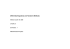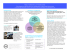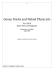# NCCTM Central Region Conference - NC Mathematics

## Transcription

NCCTM Central Region Conference - NC Mathematics
```2012
NCCTM
Central Region Conference
Robin Barbour
[email protected]
Hong Kong / US Data
highest scores in the
most recent TIMMS.
•  Hong Kong students
were taught 45% of
objectives tested.
•  Hong Kong students
outperformed US
students on US content
that they were not
taught.
•  US students ranked
near the bottom.
•  US students
‘covered’ 80% of
TIMMS content.
•  US students were
outperformed by
students not taught
the same
objectives.
Lessons Learned
•  Mile wide and inch deep does not work.
many specific topics are taught; rather, it
is more about ways of thinking.
•  To change students’ ways of thinking, we
must change how we teach.
Lesson Comparison
Japan and United States
The emphasis on skill acquisi1on is The emphasis on understanding is evident in the steps most common in U.S. evident in the steps of a typical Japanese classrooms lesson • Teacher instructs students in concept or skill • Teacher poses a thought provoking problem • Teacher solves example problems with class • Students and teachers explore the problem • Students prac8ce on their own while teacher assists individual students • Various students present ideas or solu8ons to the class • Teacher summarizes the class solu8ons • Students solve similar problems 4
Types of Math Problems Presented
How Teachers Implemented Making
Connections Math Problems
Points to Ponder
•  What is the goal of teaching mathematics?
What am I really trying to accomplish in
my classroom?
•  Does my lesson plan emphasize
mathematical thinking and process, or is
the goal to demonstrate procedures and
Points to Ponder
•  Is there a place in my classroom for
lecture? Do I balance my teaching
strategies?
•  Do I help my students find connections
between big ideas in mathematics?
•  What type of seatwork do my students do?
•  What kinds of questions do I ask in my
classes?
Points to Ponder
•  Do I tend to encourage students to find
multiple ways to solve the same problem?
•  What do I do when students get frustrated
or confused?
•  What do I do when a student makes a
mistake?
Hoffman, Lauren R. and Brahier, Daniel J., Improving the Planning and Teaching of
Mathematics by Reflecting on Research. Mathematics Teaching in the Middle School. VOL.
13, NO. 76, March 2008
www.corestandards.org
Standards for Mathematical Practices
1.  Make sense of problems and persevere in solving
them
2.  Reason abstractly and quantitatively
3.  Construct viable arguments and critique the
reasoning of others
4.  Model with mathematics
5.  Use appropriate tools strategically
6.  Attend to precision
7.  Look for and make use of structure
8.  Look for and express regularity in repeated reasoning
S
T
U
D
E
N
T
E
N
G
A
G
E
M
E
N
T
Mathematics Claims
The Smarter Balanced Assessment Consortium
has released a document outlining four
students can do.
The claims are a synthesis of the Standards for
Mathematical Practice, and form the guiding
principles to be used in creating
assessments.
Mathematics Claim #1
Students can explain and apply
mathematical concepts and
carry out mathematical
procedures with precision and
fluency.
Mathematics Claim #2
Students can frame and solve a
range of complex problems in
pure and applied mathematics.
Mathematics Claim #3
Students can clearly and
precisely construct viable
arguments to support their own
reasoning and to critique the
reasoning of others.
Mathematics Claim #4
Students can analyze complex,
real-world scenarios and can use
mathematical models to interpret
and solve problems.
Which of the following represents 2/5?
a.
b.
c.
d.
For numbers 1a – 1d, state whether or not each
figure has 2/5 of its whole shaded.
1a.
ο Yes
ο No
ο Yes
ο No
1c.
ο Yes
ο No
1d.
ο Yes
ο No
1b.
Scoring Rubric
Responses to this item will receive 0 – 2 points, based
upon the following:
2 points: YNYN
1 point: YNNN, YYNN, YYYN
0 point: YYYY, YNNY, NNNN, NNYY, NYYN,
NYNN, NYYY, NYNY, NNYN, NNNY, YYNY,
YNYY
For further information…
Smarter Balanced Assessment Consortium
http://www.k12.wa.us/smarter/
K – 8 Domains"
Domains
K
1
2
3
4
5
6
7
8
Counting and Cardinality
Operations and Algebraic Thinking
Number and Operations in Base Ten
Measurement and Data
Geometry
Number and Operations - Fractions
Ratios and Proportional Relationships
The Number System
Expressions and Equations
Statistics and Probability
Functions
3/1/12 • page 27
Standards"
High School Standards Notation
Perform operations on matrices and use matrices in applications. 6. (+) Use matrices to represent and manipulate data, e.g., to represent
payoffs of incidence relationship in a network. 11. Explain why the x-­coordinates of the points where the graphs of the
equations y = f(x) and y =g(x intersect are the solutions of the equations f(x)
= g(x); Bind the solutions approximately, e.g., using technology to graph the
functions, make tables of values, or Bind successive approximations. Include
cases where f(x) and/or g(x) are linear, polynomial, rational, absolute value,
exponential, and logarithmic functions.★ High School Themes
•
•
•
•
•
•
Number and Quantity
Algebra
Functions
Modeling
Geometry
Statistics and Probability
Unpacked Content
3/1/12 • page 31
www.ncdpi.wikispaces.net
3/1/12
32
Four Teacher-Friendly Postulates
for Thriving In a Sea of Change
Steve Leinwand
1.  We are being asked to teach in distinctly different
ways from how we were taught.
2. The traditional curriculum was designed to meet
societal needs that no longer exist.
3.  It is unreasonable to ask a professional to
change much more than 10 percent a year, it is
unprofessional to change by much less than 10
percent a year.
4. If you don’t feel inadequate you are probably not
doing the job.
Contact Information
Robin Barbour
Middle School Mathematics
[email protected]
Johannah Maynor
High School Mathematics
[email protected]
Website:
www.ncdpi.wikispaces.net
QUESTIONS
```

### MAT.HS.CR.1.00FIF.L.614 HS Mathematics Sample CR Item C1 TL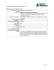### Kolams and Mathematical Thinking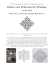### MAT.HS.CR.2.0STCP.D.070 HS Mathematics Sample CR Item Claim 2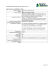### MAT.HS.CR.2.0ASSE.A.005 HS Mathematics Sample ER Item Claim 2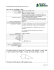### Sonia Kovalevsky Math Day Poster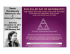### Mathematics Word Wall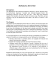### The concept of treatment in thematic philately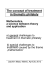### Author - Center for Innovation in Education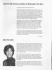### this PDF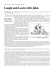### here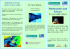### Spa, Petaling Jaya, Malaysia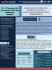### Drawing Math Stories: Linking Language, Art, and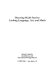### MathOverflow - Department of Math/CS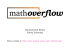### Mathematical Modeling as a Means to Demonstrate the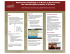### Tracy Curran, Cloneen NS - INTO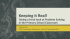### Presentation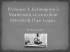### Counting to Calculus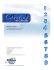### Associate Degree in Why study Mathematics? Mathematics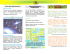### program overview - Art of Problem Solving Foundation### Differential Equations and Transform Methods Module code: EE 206